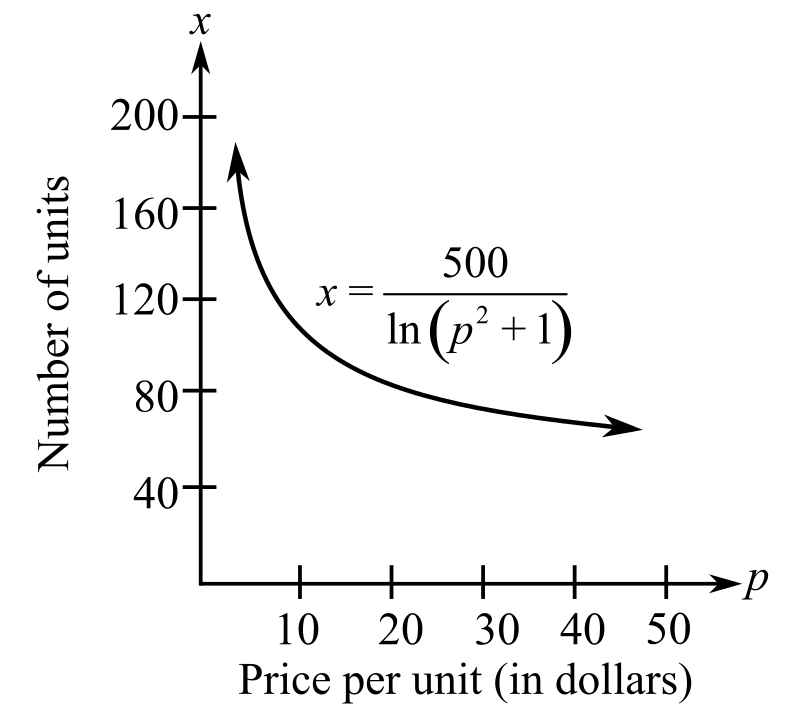Chapter 4.5, Problem 76E### Calculus: An Applied Approach (Min...

10th Edition
Ron Larson
ISBN: 9781305860919

#### Solutions

Chapter
Section### Calculus: An Applied Approach (Min...

10th Edition
Ron Larson
ISBN: 9781305860919
Textbook Problem
1 views

# Demand Solve the demand function in Exercise 74 for p. Use the result to find dp/dx. Then find the rate of change when p = $10. What is the relationship between this derivative and dx/dp? To determine To calculate: The value of demand function for p by using the result dpdx for the demand function which is approximated by x=500ln(p2+1) and to explain the same for the change of a price of$10 if the demand function is as follows:Explanation

Given information:

The provided function is x=500ln(p2+1) and the graph is,

Formula used:

The derivative of e raised to a function is ddxeu=eududx.

Constant multiple rule of derivative of function f(x) is f(cx)=cf(x) where, c is constant.

The derivative of function f(x)=un using the chain rule is:

f(x)=ddx(un)=nun1dudx

Where, u is the function of x.

Calculation:

Consider the function x=500ln(p2+1),

Rewrite the provided function with rational exponent,

x=500ln(p2+1)ln(p2+1)=500x(p2+1)=e500xp2=e500x1

Further calculate as,

p=(e500x1)12

Apply the exponential function of derivative to the function p=(e500x1)12,

dpdx=ddp(e500x1)12=12(e500x1)12ddx(

### Still sussing out bartleby?

Check out a sample textbook solution.

See a sample solution

#### The Solution to Your Study Problems

Bartleby provides explanations to thousands of textbook problems written by our experts, many with advanced degrees!

Get Started

#### Find more solutions based on key concepts